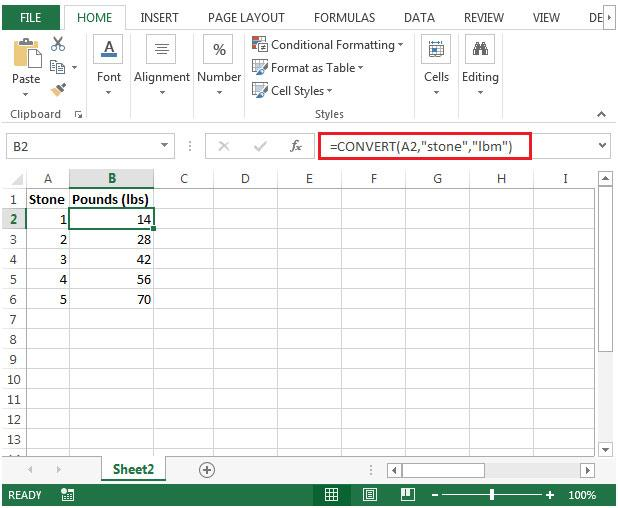How to Convert Stones to LBS (Pounds) in Microsoft Excel

In this article, we will learn how to convert stone to lbs (Pounds) in Microsoft Excel.

Stone is a measuring unit equal to 6.35 kg Approx. or 14 pounds. It is used in Britain and Ireland for measuring human body weight.Symbol of Stone is st.

The pound is a unit of mass or weight in a number of different systems, including English units. The most commonly used pound today is the international avoirdupois pound. The international avoirdupois pound is equal to exactly 453.59237 grams.Symbol of Pound is lb.

• 1 Stone = 14 Pound
• 1 Stone = 6.35 kg

Conversion of measurement can be easily done in Excel. Excel gives you default and customized measurement conversion function.

To convert Stones to Pounds, we will use the Convert Function.

CONVERT function converts the number from one measurement system to another.

Syntax:

 =CONVERT (number, from_unit, to_unit)

Here some data measured in stones which we want to convert to pound.Type  the formula in B2 cell.

 =CONVERT(A2,” Stone”,”lbm”)

Copy the formula in other cells, select the cells taking the first cell where the formula is already applied, use shortcut key Ctrl + D.As you can see you can convert stone to lbs now using the above function. We can convert kg to pound using kg to stone and then stone to pound. And to convert pound to stone we can use from_unit as lbs and to_unit as stones.

Hope you learned how to use conversion using CONVERT function in Excel. Excel lets you perform in Excel 2013 and 2010. Explore more articles on conversion here. Please let us know if you any unresolved query. We will help you.

Related Articles:

How to convert Stone to lbs weight in Excel

How to convert minutes to hours and minutes in Excel

How to Convert Date and Time from GMT to CST in Excel

Popular Articles:

50 Excel Shortcut to Increase Your Productivity

How to use the VLOOKUP Function in Excel

How to use the COUNTIF function in Excel 2016

How to use the SUMIF Function in Excel

Terms and Conditions of use

The applications/code on this site are distributed as is and without warranties or liability. In no event shall the owner of the copyrights, or the authors of the applications/code be liable for any loss of profit, any problems or any damage resulting from the use or evaluation of the applications/code.